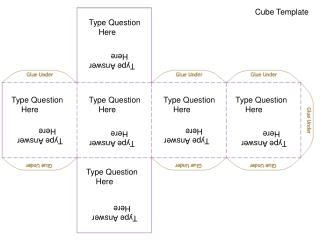DownloadDownload PresentationType Question Here

Type Question Here

Télécharger la présentationType Question Here

- - - - - - - - - - - - - - - - - - - - - - - - - - - E N D - - - - - - - - - - - - - - - - - - - - - - - - - - -
Presentation Transcript

1. Cube Template Type Question Here Type Answer Here Type Question Here Type Question Here Type Question Here Type Question Here Type Answer Here Type Answer Here Type Answer Here Type Answer Here Type Question Here Type Answer Here